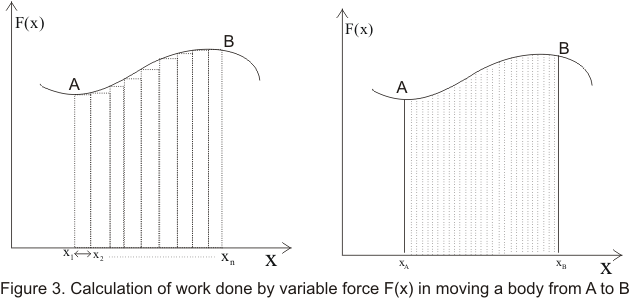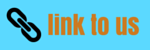# Mechanical Energy|Kinetic Energy

## (3) Work done by variable force

• So far we have defined work done by a force which is constant in both magnitude and direction.
• However, work can be done by forces that varies in magnitude and direction during the displacement of the body on which it acts.
• For simplicity consider the direction of force acting on the body to be along x-axis also consider the force F(x) is some known function of position x
• Now total displacement or path of the body can be decomposed into number of small intervals Δx such that with in each interval force F(x) can be considered to be approximately constant as shown below in the figure• Workdone in moving the body from x1 to x2 is given by
ΔW=F(x1)Δx1 where Δx1=x2-x1
• Total workdone in moving the body from point A to point B
W=F(x1)Δx1 +F(x2)Δx2 +F(x3)Δx3 .....+F(xn)Δxn
W=Σ F(xi)Δxi where i=1 to i=n           (4)
Where Σ is the symbol of summation
• Summation in equation 4 is equal to shaded area in figure 3(a) .More accuracy of results can be obtained by making these interval infintesimally smaller
• We get the exact value of workdone by making each interval so much small such that #916;x-> 0 which means curved path being decomposed into infinte number of line segment i.e
W= LimΔx-> 0Σ F(xi)Δxi           (5)
W =∫ F(x)dx with in the limits xA and xB
Where ∫ F(x)dx is the intregal of F(x) w.r.t x between the limits xA and xB and intregal can be evaluated using methods of calculus if F(x) of x is known
• Instead of x if the force also acts along y and z axis i.e direction of force keeps on changing then workdone bu such a force is given by

W=∫ F(r)dr with in the limits rA and rB           (6)
Where F(r)=F(x)i +F(y)j+ F(z)k
and dr=dxi +dyj+ dzk
Here F(x),F(y) and F(z) are rectangular components of the force along x ,y and z axis .Similary dx,dy and dz are rectangular components of displacement along
x ,y and z axis

## (4) Mechanical Energy

• we already have an idea that energy is associated closely with work and we have defined energy of a body as the capacity of the body to do work
• In dynamics body can do work either due to its motion ,due to its position or both due to its motion and position
• Ability of a body to do work due to its motion is called kinetic energy for example piston of a locomotive is capable of doing of work
• Ability of a body to do work due to its position or shape is called potential energy For example workdone by a body due to gravity above surface of earth
• Sum of kinetic energy and Potential energy of body is known as its mechanical energy
Thus
M.E=K.E+P.E

### (5) Kinetic energy

• Kinetic energy is the energy possesed by the body by virtue of its motion
• Body moving with greater velocity would posses greater K.E in comparison of the body moving with slower velocity
• Consider a body of mass m moving under the influenece of constant force F.From newton's second law of motion
F=ma
Where a is the acceleration of the body
• If due to this acceleration a,velocity of the body increases from v1 to v2 during the displacement d then from equation of motion with constant acceleration we have
a=v22 -v12/2d Using this acceleration in Newton's second law of motion
we have
F=m(v22 -v12)/2d
or
Fd=m(v22 -v12)/2
or
Fd=mv22/2 -mv12/2           (7)
We know that Fd is the workdone by the force F in moving body through distance d
• In equation(7),quantity on the right hand side mv2/2 is called the kinetic energy of the body
Thus
K=mv2/2
• Finally we can define KE of the body as one half of the product of mass of the body and the square of its speed
• Thus we see that quantity (mv2/2) arises purely becuase of the motion of the body
• In equation 7 quantity
K2=mv22/2
is the final KE of the body and
K1=mv12/2
is the initial KE of the body .Thus equation 7 becomes
W=K2-K1=ΔK           (9)
• Where ΔK is the change in KE.Hence from equation (9) ,we see that workdone by a force on a body is equal to the change in kinetic energy of the body
• Kinetic energy like work is a scalar quantity
• Unit of KE is same as that of work i.e Joule
• If there are number of forces acting on a body then we can find the resultant force ,which is the vector sum of all the forces and then find the workdone on the body
• Again equation (9) is a generalized result relating change in KE of the object and the net workdone on it.This equation can be summerized as
Kf=Ki+W           (10)
which says that kinetic energy after net workdone is equal to the KE before net work plus network done.Above statement is also known as work-kinetic energy theorem of particles
• Work energy theorem holds for both positive and negative workdone.if the workdone is positive then final KE increases by amount of the work and if workdone is negative then final KE decreases by the amount of workdone